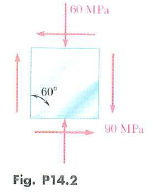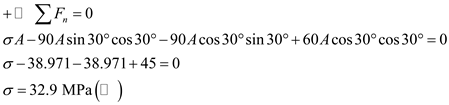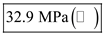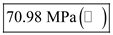# For the given slate of stress, determine the normal and shearing stresses exerted on the oblique lace of the shaded triangular element shown. Use a method of analysis based on the equilibrium of that element as was done in the derivations of Sec. 14.2.

Question-AnswerCategory: Strength of MaterialsFor the given slate of stress, determine the normal and shearing stresses exerted on the oblique lace of the shaded triangular element shown. Use a method of analysis based on the equilibrium of that element as was done in the derivations of Sec. 14.2.

For the given slate of stress, determine the normal and shearing stresses exerted on the oblique lace of the shaded triangular element shown. Use a method of analysis based on the equilibrium of that element as was done in the derivations of Sec. 14.2.Step: 1

Represent the state of stress element.Step: 2

Consider the area of the element.Step: 3

Consider the loads acting on the element.Step: 4

Consider the equilibrium of forces along the normal direction of the plane.There,is the area of the oblique plane.
Therefore, the normal stress exerted on the oblique face is.

Step: 5

Consider the equilibrium of forces along the plane.Therefore, the shear stress exerted on the oblique face is.